# Ratio and Proportion Questions for Competitive Exams

Ratio and Proportion Questions for Competitive Exams in English. Important Mats MCQ practice question and answer from the previous year exam paper of SSC CGL, CHSL, CPO, NRA CET, UPSSSC, Bank, UPSC NDA, CDS etc.

### Questions on Basic Concept of Ratio and Proportion

Q.1: What must be added to each term of the ratio 2 : 5 so that it may equal to 5 : 6?
a) 65
b) 78
c) 13
d) 12

Ans : c) 13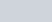$\frac {2+x}{5+x} = \frac 56$
= 12 + 6x = 25 +5x
=> x= 13

Q.2: In a cricket match there are three types of tickets say A, B and C each costing ₹ 1000,₹ 500 and ₹ 200 respectively. The ratio of the ticket sold of category A, B and C is 3 : 2 : 5. If the total collection from selling the tickets is ₹ 2.5 crore. Find the total number of tickets sold.
a) 50000
B) 40000
c) 45000
d) 60000

Ans : 50000
Sold tickets of A,B,C categories are 3x, 2x, and 5x respectively.
Total Collection =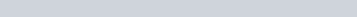$(3x \times 1000)+(2x \times 500)+(5x \times 200) = 25000000$
5000x = 25000000
x = 5000
Total sold tickets = 3x+2x+5x = 10x = 50000

Q.3: If A : B = 7 : 9 and B : C = 3 : 5, then A : B : C is equal to
a) 7 : 9 : 5
b) 21: 35: 45
c) 7 : 9 : 15
d) 7 : 3 : 15

Ans : c) 7 : 9 : 15
A : B = 7 : 9 and B : C = 3 : 5 =9 : 15 (multiply by 3)
A:B:C = 7 : 9 : 15 ( B= 9 in both ration)

Q.4: The ratio of the length of a school ground to its width is 5 : 2. If the width is 40 m, then the length is
a) 200 m
b) 100 m
c) 50 m
d) 80 m

Ans : b) 100 m
Length : Breadth = 5 : 2,
Length : 40 = 5 : 2,
L x 2 = 40 x 5, L = 100 m

Q.5: The ratio of boys and girls in a college is 5 : 3. If 50 boys leave the college and 50 girls join the college, the ratio becomes 9 : 7. The number of boys in the college is :
a) 300
b) 400
c) 500
d) 600

Ans : c) 500
5x -50 : 3x+50 = 9 :7
35x-350 = 27x+450
x = 100
Boys = 5 x = 500

Q.6: In a school, the ratio of boys to girls is 4 : 3 and the ratio of girls to teachers is 8 : 1. The ratio of students to teachers is
a) 56 : 3
b) 55 : 1
c) 49 : 3
d) 56 : 1

Ans : a) 56 : 3
Boys : Girls = 4 : 3 = 32 : 24
Girls : Teachers = 8 : 1 = 24 : 3
Boys : Girls : Teachers = 32 : 24 : 3
Students (Boys + girls) : Teachers = (32+24) : 3 = 56 : 3

Q.7: The ratio 43.5 : 25 is the same as :
a) 4 : 1
b) 2 : 1
c) 1 : 2
d) 1 : 4

Ans : a) 4 : 1
43.5 : 25 = 43 x 40.5 : 25
= 64 x 2 : 32 = 4 : 1

Q.8: If ₹ 1000 is divided between A and B in the ratio 3 : 2, then A will receive :
a) ₹ 400
b) ₹ 500
c) ₹ 600
d) ₹ 800

Ans : c) ₹ 600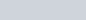$\frac { 3 \times 1000}{5} = 600$

### Ratio and Proportion : Fractions Questions

Q. 9: If x : y = 3 : 4 and y : z = 3 : 4, then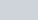$\frac {x+y+z}{3z}$ is equal to:
a)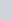$\frac {13}{27}$
b)$\frac {1}{2}$
c)$\frac {73}{84}$
d)$\frac {37}{48}$

Ans : d)$\frac {37}{48}$
x : y = 3 : 4 = 9: 12 and y : z = 3 : 4 = 12 : 16,
x : y : z = 9 : 12 : 16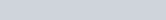$\frac {x+y+z}{3z} = \frac {9k+12k+16k}{3\times 16k} = \frac {37}{48}$

Q.10: If x : y = 3 : 4, then the value of (4x− y) : (2x + 3y) is
a) 4 : 9
b) 8 : 9
c) 4 : 3
d) 8 : 3

Ans : a) 4 : 9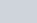$\frac xy = \frac 34$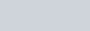$\frac {4x - y} {2x+3y} = \frac {4\frac {x}{y} - 1}{2\frac{x}{y} + 3 }$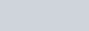$= \frac {4 \times \frac {3}{4} - 1}{2 \times \frac{3}{4} + 3 } = \frac 49$

### Ration and Proration : Percentage Questions

Q.11: There is a ratio of 5 : 4 between two numbers. If 40 per cent of the first is 12, then 50% of the second number is :
a) 18
b) 24
c) 12
d) 20

Ans : c) 12
Number are 5x and 4x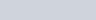$5x \times \frac{40}{100} = 12$
x = 6
Second number is 4x = 24
50% of 24 = 12

### Ratio and Proportion Questions Based on Age for Competitive Exams

Q.12: The ratio of ages of two persons is 5 : 9 and the age of one of them is greater than the other by 40 years. The sum of their ages in year is :
a) 180
b) 140
c) 150
d) 160

Ans : b) 140
Let age are 5x and 9x
9x – 5x = 4x = 40 , x =10
Sum of age = 5x + 9x = 14x = 140

Q.13: The ratio of the ages of A and B at present is 3 : 1. Four years earlier the ratio was 4 : 1. The present age of A is :
a) 48 years
b) 40 years
c) 36 years
d) 32 years

Ans : c) 36 years
Present age is 3x and x
4 year ago :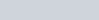$\frac {3x-4}{x-4} = frac 51$
x =12
A present age is 3x = 36

Q.14: The average age of boys in the class is twice the number of girls in the class. The ratio of boys and girls in the class of 50 is 4 : 1. The sum of the ages (in years) of the boys in the class is :
a) 2000
b) 2500
c) 800
d) 400

Ans : c) 800
Boys -40, Girls -10
Average Age of boys = 20
Total Age of Boys = 20 x40 = 800

Q.15: The ratio of ages of two boys is 5 : 6. After two years the ratio will be 7 : 8. The ratio of their age after 12 years will be :
a) 22 : 24
b) 15 : 16
c) 17 : 18
d) 11 : 12

Ans : c) 17 : 18
Ratio after 2 years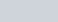$\frac {5x + 2}{6x+2} = \frac78$
= 42x + 14 = 40x +16, x = 1
Ration after 12 years
5x+12 : 6x+12 = 17 : 18

Q.16: The ratio of the age of a father to that of his son is 5 : 2. If the product of their ages in years is 1000, then the father’s age (in years) after 10 years will be:
a) 50
b) 60
c) 80
d) 100

Ans : b) 60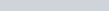$5x \times 2x = 1000$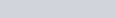$x^2 =100, x = 10$
Father age after 10 years
5x + 10 = 50 + 10 = 60

Q.17: Harsha is 40 years old and Ritu is 60 years old. How many years ago was the ratio of their ages 3 : 5 ?
a) 10 years
b) 20 years
c) 37 years
d) 5 years

Ans : a) 10 years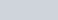$\frac {40-x}{60-x} = \frac 35$
200 – 5x = 180 – 3x,
x = 10 years

### Ratio and Proportion Questions : Addition, Difference and Product

Q. 18: Two numbers are in ratio 5 : 8. If their difference is 48, then the smaller number is :
a) 80
b) 96
c) 128
d) 64

Ans : a) 80
8x – 5x =48, x= 16
Smaller number is 5x = 80

Q.19: If A and B are in the ratio 4 : 5 and the difference of their squares is 81, what is the value of A?
a) 45
b) 12
c) 36
d) 15

Ans : b) 12
(5x)2 – (4x)2 = 81
25x2 – 16x2 =81
x2 = 9, x= 3
A = 4x = 12

Q.20: The ratio of three positive numbers is 2 : 3 : 5 and the sum of their squares is 608. The three numbers are
a) 2,3,5
b) 10,15,25
c) 8,12,20
d) 4,6,10

Ans : c) 8,12,20
(2x)2 + (3x)2 + (5x)2 = 608
38x2 = 608
x2 =16, x=4
Number are 8,12,20

Q.21: If the square of the sum of two numbers is equal to 4 times of their product, then the ratio of these numbers is :
a) 2 : 1
b) 1 : 3
c) 1 : 1
d) 1 : 2

Ans : c) 1 : 1
(x + y)2 = 4xy
x2 + y2 + 2xy – 4xy = 0
x2 + y2 – 2xy = 0
(x – y)2= 0, x = y
x : y = 1 : 1

Q.22: The ratio of number of balls in bags x, y is 2 : 3. Five balls are taken from bag y and are dropped in bag x. The number of balls is equal in each bag now. The number of balls in each bag now is :
a) 45
b) 20
c) 30
d) 25

Ans : b) 20
Number of balls in bag x and y respectively = 2a and 3a
3a – 5 = 21 + 3, a = 8
Total number of balls = 5 a = 40
Balls in each bag = 20

Q.23: Which number when added to each of the numbers 6, 7, 15, 17 will make the resulting numbers proportional?
a) 6
b) 5
c) 4
d) 3

Ans : d) 3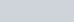$\frac { 6+x}{7+x} = \frac{15+x}{17+x}$
=> 102+17x + 6x +x2 = 105 +7x +15x +x2
=> 23x – 22x = 105 -102, => x = 3

Q.24: In a 45 Liters mixture of milk and water, the ratio of the milk to water is 2 : 1. When some quantity of water is added to the mixture, this ratio becomes 1 : 2. The quantity of water added is :
a) 10 Liters
b) 21 Liters
c) 35 Liters
d) 45 Liters

Ans : d) 45 Liters
Milk : Water : 2 : 1 = 30: 15
Let x Ltrs of water is added and ratio become 1:2
30 : (15+x) = 1 : 2
=> 15 + x = 60, x =45 Liters

### Ratio and Proportion Questions : LCM and HCF

Q.25: Two numbers are in the ratio 3 : 5 and their LCM is 225. The smaller number is :
a) 45
b) 60
c) 75
d) 90

Ans : a) 45
Number are 3x and 5 x
LCM = 15 x
15 x = 225, x = 15
Smaller number = 3x = 45

### Ratio and Proportion Questions for Competitive Exams: Coins and Rupees

Q.26: A box contains 280 coins of denomination carrying 1 rupee, 50 paise and 25 paise. The values of each kind of the coins are in the ratio of 8 : 4 : 3. Then the number of 50 paise coins is :
a) 70
b) 60
c) 80
d) 90

Ans : c) 80
Ratio of the values of one rupee, 50 paise and 25 paise coins = 8 : 4 : 3
Ratio of their number = 8 : 4 x 2 : 3 x 4 = 2:2:3
Sum of Ratio : 2+2+3=7
number of 50 paise coins =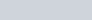$280 \times \frac 27 = 80$

Q.27: A bag contains three types of coins. One rupee-coins, 50 paisa-coins and 25 paisa coins totaling 175 coins. If the total value of the coins of each kind be the same, the total amount in the bag is :
a) ₹ 75
b) ₹ 175
c) ₹ 300
d) ₹ 126

Ans : a) ₹ 75
Let number of One rupee coins are A
Value of each kind of coins is same, hence 50 paisa coins 2A and 25 paisa coins 4A.
A + 2A + 4A = 7A = 175, A = 25
Total Amount in bag = 3A = 75

### Ratio and Proportion : Shares and Partners

Q.28: In a business A and C invested amounts in the ratio of 2 : 1, whereas A and B invested amounts in the ratio of 3 : 2. If their annual profit be ₹ 1,57,300, then B’s share in the profit is :
a) ₹ 24200
b) ₹ 48000
c) ₹ 36300
d) ₹ 48400

B Share =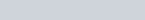$157300 \times \frac {4}{13} = 48400$